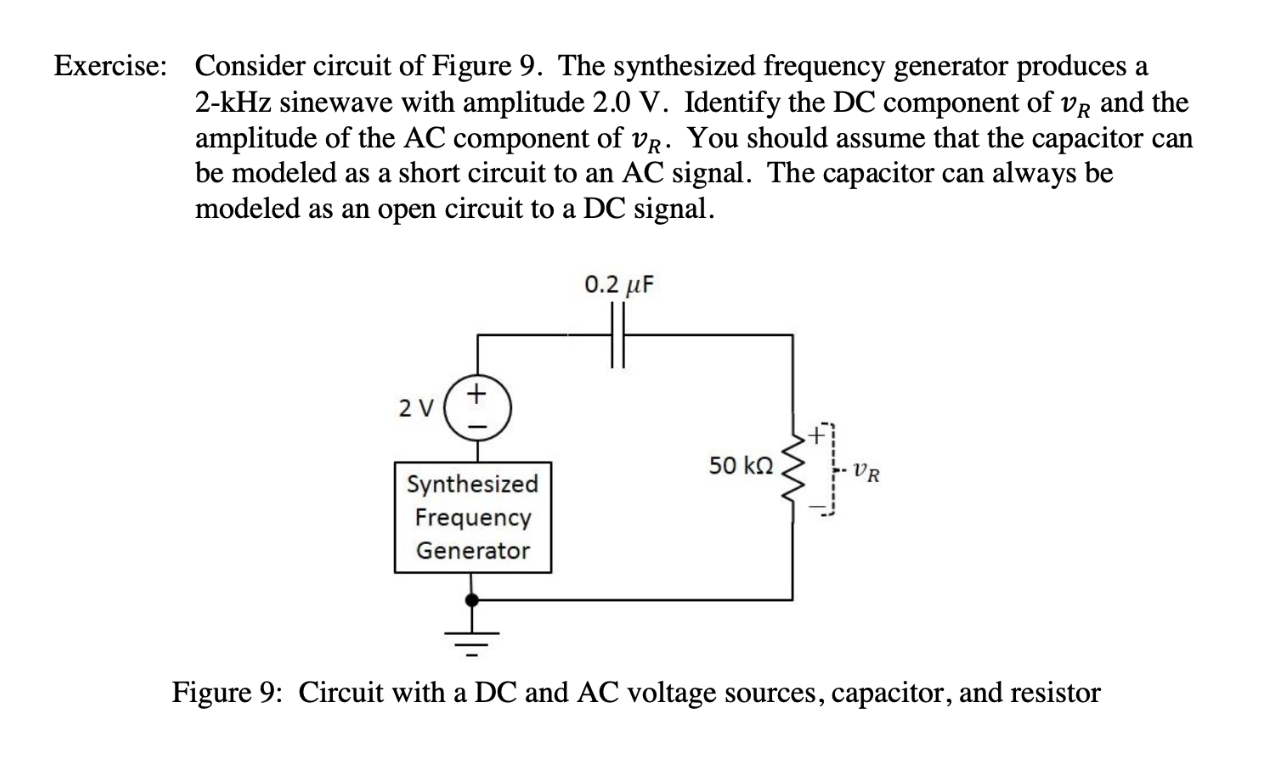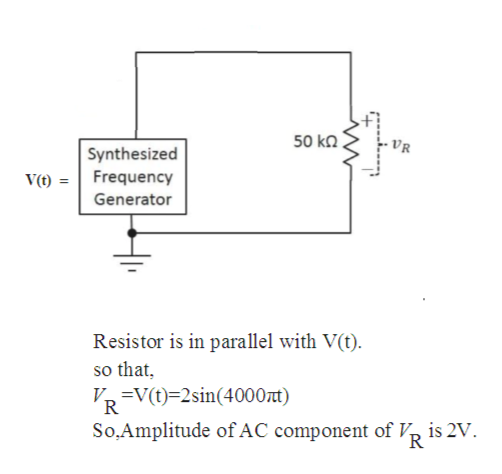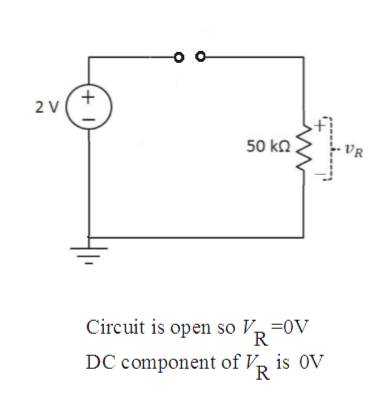# Consider circuit of Figure 9. The synthesized frequency generator produces2-kHz sinewave with amplitude 2.0 V. Identify the DC component of vR and theamplitude of the AC component of vR. You should assume that the capacitorbe modeled as a short circuit to an AC signal. The capacitormodeled as an open circuit to a DC signalExercise:аcanalways becan0.2 uF2 V50 kQVRSynthesizedFrequencyGeneratorFigure 9: Circuit with a DC and AC voltage sources, capacitor, and resistorw

Question
2 viewshelp_outlineImage TranscriptioncloseConsider circuit of Figure 9. The synthesized frequency generator produces 2-kHz sinewave with amplitude 2.0 V. Identify the DC component of vR and the amplitude of the AC component of vR. You should assume that the capacitor be modeled as a short circuit to an AC signal. The capacitor modeled as an open circuit to a DC signal Exercise: а can always be can 0.2 uF 2 V 50 kQ VR Synthesized Frequency Generator Figure 9: Circuit with a DC and AC voltage sources, capacitor, and resistor w fullscreen
check_circle

star
star
star
star
star
1 Rating
Step 1

Assume, output of synthesized frequency generator is V(t).

Synthesized frequency generator produces a sinewave signal having amplitude 2V and frequency 2KHz.

So,

Step 2

In given circuit, AC and DC both voltage source is present so that using superposition Theorem in circuit.

Superposition theorem states that in a linear, bilateral ,active network if two or more than two independent sources are present then response in any branch of network would be algebraic sum of all responses produced by each independent source acting individually and other voltage source should short circuited and current source should open circuited.

Now, First take A.C source as it is and D.C source shorted.

Capacitor is also shorted because it is mentioned in question that capacitor should be shorted for A.C signal.help_outlineImage Transcriptionclose50 kn VR Synthesized V(t) =Frequency Generator Resistor is in parallel with V(t) so that V=V(t)=2sin(4000nt) So.Amplitude ofAC component of V is 2V. fullscreen
Step 3

Now, Take D.C source as it is and A.C source shorted.

Capacitor is open because it is men...help_outlineImage Transcriptionclose2 V 50 k VR Circuit is open so V -0V DC component of V, is OV fullscreen

### Want to see the full answer?

See Solution

#### Want to see this answer and more?

Solutions are written by subject experts who are available 24/7. Questions are typically answered within 1 hour.*

See Solution
*Response times may vary by subject and question.
Tagged in

### Electrical Engineering